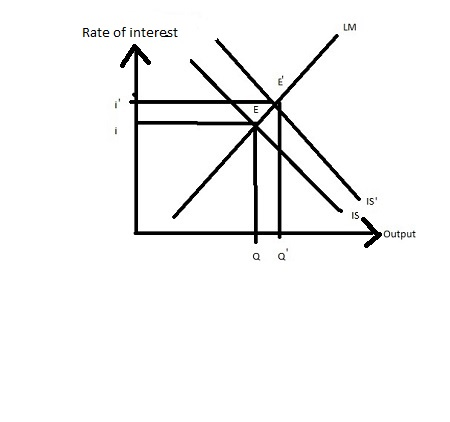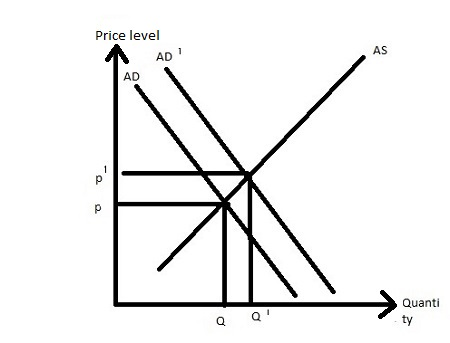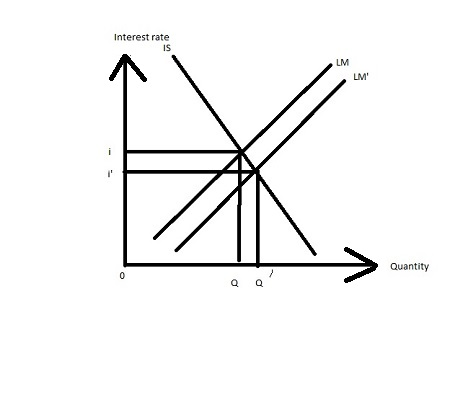# For which of the following would you use dynamic aggregate supply and demand analysis? The...

## Question:

For which of the following would you use dynamic aggregate supply and demand analysis?

The alternative would be static aggregate supply and demand analysis. There might be more than one answer.

1) If government purchases increase, does real GDP grow or shrink

2) The economy is currently growing at 2%. Would the growth rate be more if the Fed lowered the federal funds rate?

3) Does the price level rise or fall if taxes fall?

4) The current inflation is about 1%. If taxes fell, would the inflation rate rise?

## Aggregate demand and Aggregate Supply:

As the price level rises then the total quantity of all good demanded falls and as the price level falls then the total quantity of all good demanded rises shows that the aggregate demand curve is downward sloping.

As the price level rises then the total quantity of all good supplied also rises and as the price level falls then the total quantity of all good supplied also falls shows that the aggregate supply curve is upward sloping.

## Answer and Explanation:

1) As the government purchase increases then IS curve shifts rightward which increases the rate of interest in such a way that the aggregate demand curve shifts rightward with the same aggregate supply curve such that both price level and output or real GDP increases.2) If the federal reserve reduces the federal funds rate then the money supply increases which reduces the rate of interest in such a way that the investment increases that cause the aggregate demand curve shift rightward which increases both price level and output or growth rate is more.3) As the government reduces their taxes then IS curve shifts rightward which increase the rate of interest in such a way that the aggregate demand curve shifts rightward that increases both price level or inflation rate and output.4) If taxes fell then IS curve shifts rightward which increases the rate of interest in such a way that the aggregate demand curve shifts rightward which increases both price level or inflation rate and output.#### Learn more about this topic:Aggregate Supply and Aggregate Demand (AS-AD) Model

from Economics 102: Macroeconomics

Chapter 7 / Lesson 3
58K